# SSAT Middle Level Math : Fractions

## Example Questions

← Previous 1 3 4 5 6 7 8 9 85 86

### Example Question #947 : Hspt Mathematics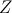is larger than 0.  Which of the following could be equal to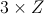?

I.II.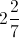III.I, II and III

I only

III only

II only

I and II

I, II and III

Explanation:

All of the answers can be divided by 3 to yield an answer larger than zero.  In fact, any positive number would be a viable answer.

### Example Question #948 : Hspt Mathematics

Express the quotient as a fraction in lowest terms: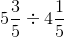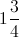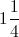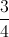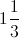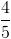Explanation:

Rewrite the mixed fractions as improper fractions, change to a multiplication by inverting the second, cross-cancel, and multiply across: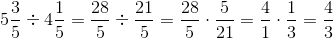### Example Question #949 : Hspt Mathematics

Express the quotient as a fraction in lowest terms: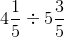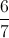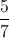Explanation:

Rewrite the mixed fractions as improper fractions, change to a multiplication by inverting the second, cross-cancel, and multiply across: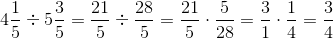### Example Question #950 : Hspt Mathematics

Evaluate: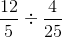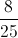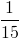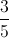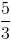Explanation:

Multiply by the reciprocal, cross-cancel, then multiply numerators and denominators: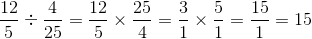### Example Question #951 : Hspt Mathematics

Evaluate: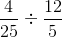Explanation:

Multiply by the reciprocal, cross-cancel, then multiply numerators and denominators: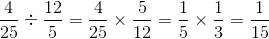### Example Question #952 : Hspt Mathematics

Evaluate: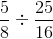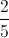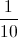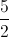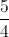Explanation:

Multiply by the reciprocal, cross-cancel, then multiply numerators and denominators: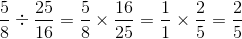### Example Question #953 : Hspt Mathematics

Evaluate: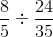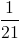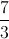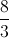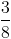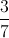Explanation:

Multiply by the reciprocal, cross-cancel, then multiply numerators and denominators: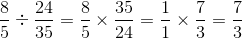### Example Question #954 : Hspt Mathematics

Evaluate: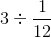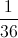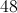Explanation: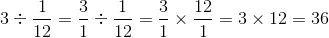### Example Question #955 : Hspt Mathematics

Evaluate: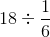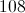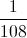Explanation: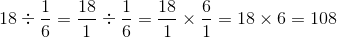### Example Question #956 : Hspt Mathematics

Evaluate: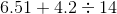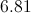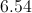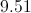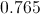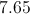Explanation:

By order of operations, divide first. Since the divisor is a whole number, divide as is: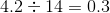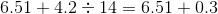Add vertically, aligning the decimal points (appending a zero to 0.3):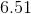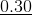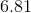← Previous 1 3 4 5 6 7 8 9 85 86

### All SSAT Middle Level Math Resources